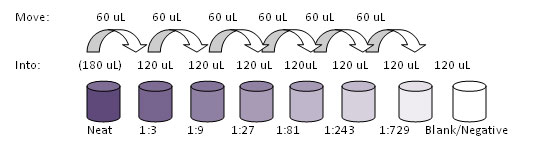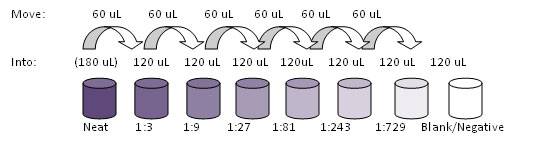# Dilutions: Explanations and Examples of Common Methods

## There are many ways of expressing concentrations and dilution.

##### Using C1V1 = C2V2

To make a fixed amount of a dilute solution from a stock solution, you can use the formula: C1V1 = C2V2 where:

• V1 = Volume of stock solution needed to make the new solution
• C1 = Concentration of stock solution
• V2 = Final volume of new solution
• C2 = Final concentration of new solution
• Example: Make 5 mL of a 0.25 M solution from a 1 M solution
• Formula: C1V1 = C2V2
• Plug values in: (V1)(1 M) = (5 mL)(0.25 M)
• Rearrange: V1 = [(5 mL)(0.25 M)] / (1 M)V1 = 1.25 mL
• Answer: Place 1.25 mL of the 1 M solution into V1-V2 = 5 mL – 1.25 mL = 3.75 mL of diluent
##### Using Dilution Factors

To make a dilute solution without calculating concentrations, you can rely on a derivation of the above formula:
(Final Volume / Solute Volume) = Dilution Factor (can also be used with mass)

This way of expressing a dilution as a ratio of the parts of solute to the total number of parts is common in biology. The dilution factor (DF) can be used alone or as the denominator of the fraction, for example, a DF of 10 means a 1:10 dilution, or 1 part solute + 9 parts diluent, for a total of 10 parts. This is different than a “dilution ratio,” which typically refers to a ratio of the parts of solute to the parts of solvent, for example, a 1:9 using the previous example. Dilution factors are related to dilution ratios in that the DF equals the parts of solvent + 1 part.

• Example: Make 300 μL of a 1:250 dilution
• Formula: Final Volume / Solute Volume = DF
• Plug values in: (300 μL) / Solute Volume = 250
• Rearrange: Solute Volume = 300 μL / 250 = 1.2 μL
• Answer: Place 1.2 μL of the stock solution into 300 μL – 1.2 μL = 298.8 μL diluent
##### Step Dilutions

If the dilution factor is larger than the final volume needed, or the amount of stock is too small to be pipetted, one or more intermediary dilutions may be required. Use the formula: Final DF = DF1 * DF2 * DF3 etc., to choose your step dilutions such that their product is the final dilution.

• Example: Make only 300 μL of a 1:1000 dilution, assuming the smallest volume you can pipette is 2 μL
• Choose step DFs: Need a total dilution factor of 1000. Let’s do a 1:10 followed by a 1:100 (10 * 100 = 1000)
• Formula: Final Volume / Solute Volume = DF
• Plug values in: (300 μL) / Solute Volume = 10
• Rearrange: Solute Volume = 300 μL / 10 = 30 μL
Answer: Perform a 1:10 dilution that makes at least 30 μL (e.g. 4 μL solute into 36 μL diluent), then move 30 μL of the mixed 1:10 into 300 μL – 3 μL = 297 μL diluent to perform the 1:100 dilution
##### Serial Dilutions

A dilution series is a succession of step dilutions, each with the same dilution factor, where the diluted material of the previous step is used to make the subsequent dilution. This is how standard curves for ELISA can be made. To make a dilution series, use the following formulas:

• Move Volume = Final Volume / (DF -1)
• Diluent Volume = Final Volume – Move Volume
• Total Mixing Volume = Diluent Volume + Move Volume
• Example 1: Make a 7-point 1:3 standard curve, starting Neat, such that you can pipette duplicates of 50 μL per well
• Calculations:
• Calculate the minimum diluent volume per step: 50 μL per well * 2 for duplicates = 100 μL minimum. Add extra volume to compensate for pipetting error, for example, 20 μL, which brings our desired Diluent Volume to 120 μL
• Calculate Move Volume: Move Volume = 120 μL / (3-1) = 60 μL
• Calculate Total Mixing Volume: Total Mixing Volume = 120 μL + 60 μL = 180 μL
• Prepare the first point of the standard curve, which is 180 μL of Neat standard
• Prepare the diluent for the rest of the points, or six aliquots of 120 μL of diluent
• Move 60 μL of the first point into the second and mix thoroughly, move 60 μL of that into the next, and so on• Example 2: Make a 7-point 1:2 standard curve, starting at a 1:5, such that you can pipette duplicates of 50 μL per well
• Calculations:
• Calculate minimum diluent volume per step: 50 μL per well * 2 for duplicates = 100 μL minimum. Add extra volume to compensate for pipetting error, for example, 20 μL, which brings our desired Diluent Volume to 120 μL
• Calculate Move Volume: Move Volume = 120 μL / (2-1) = 120 μL
• Calculate Total Mixing volume: Total Mixing Volume = 120 μL + 120 μL = 240 μL
• Calculate first point dilution volumes: you need 240 μL of a 1:5